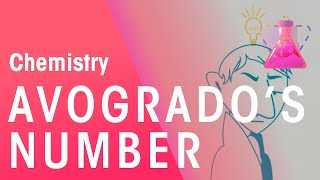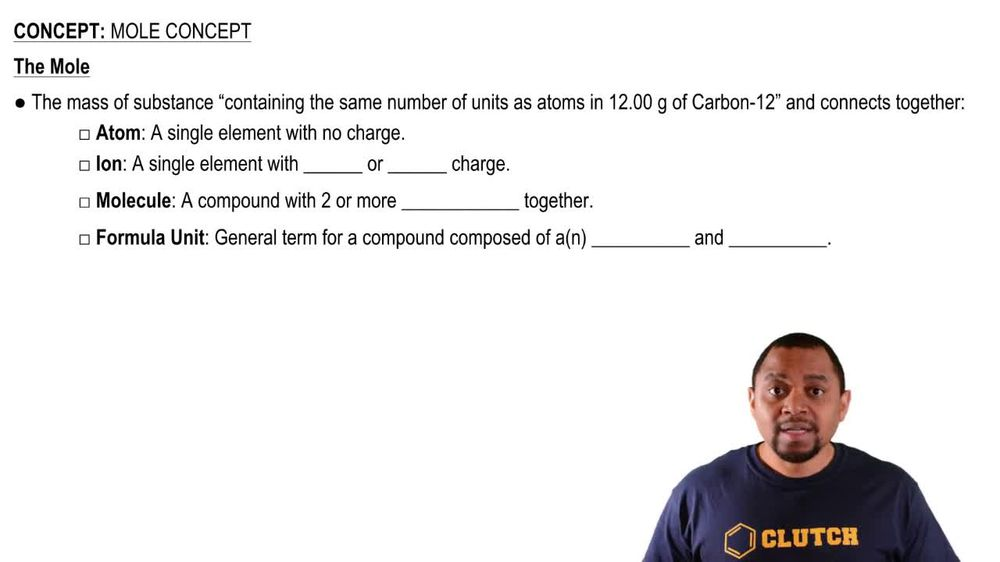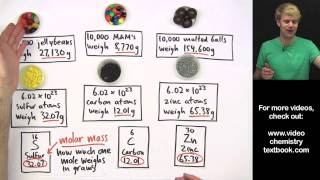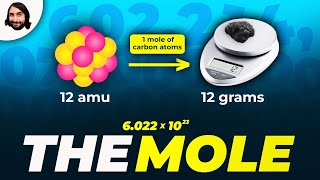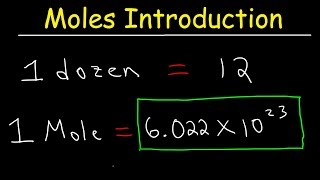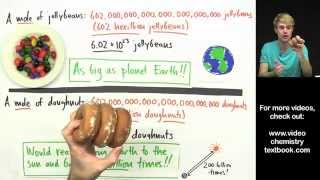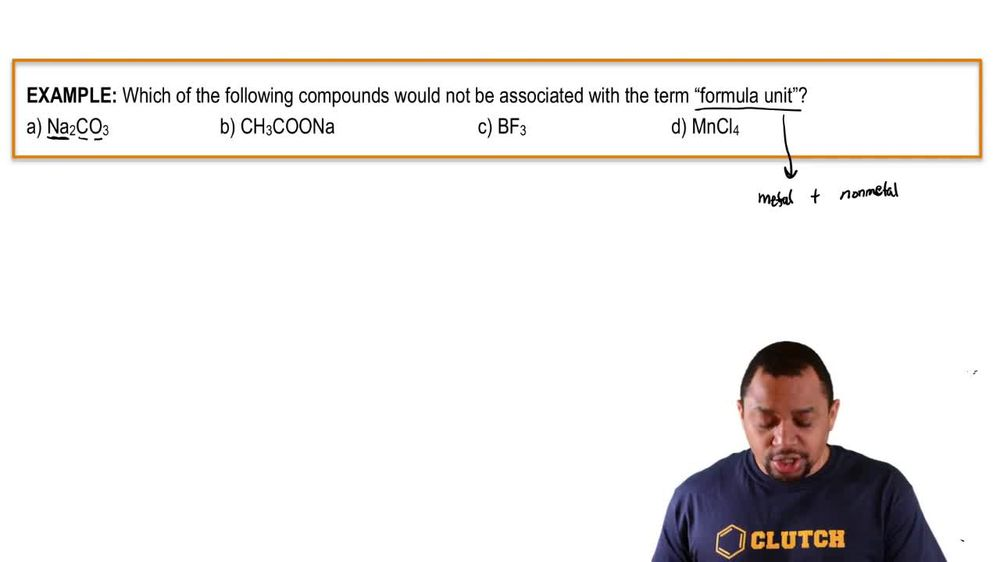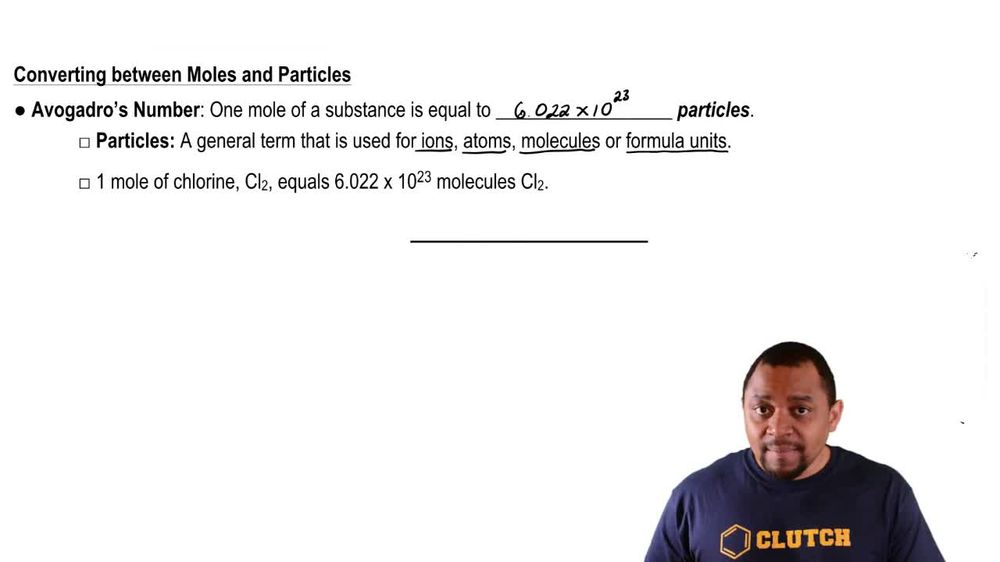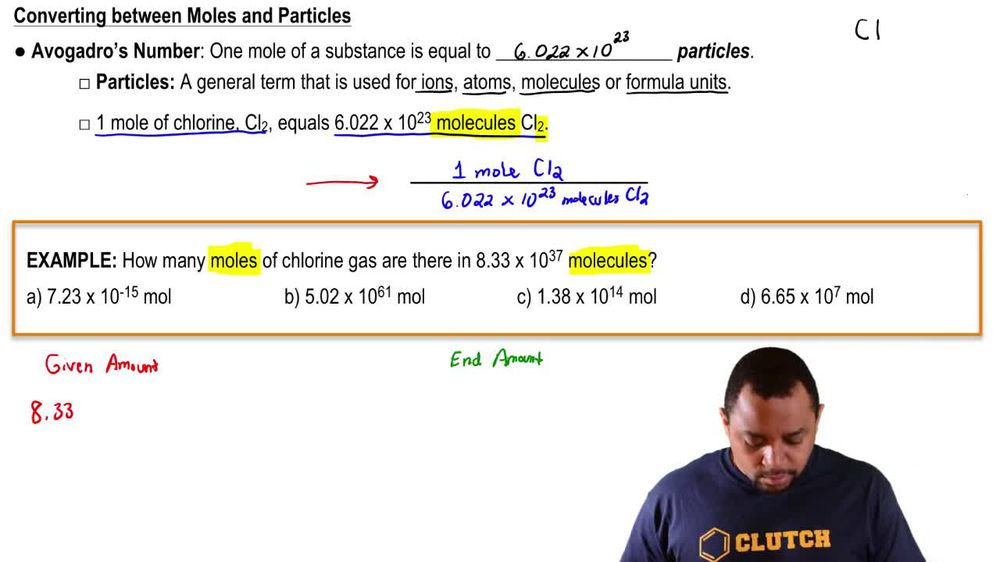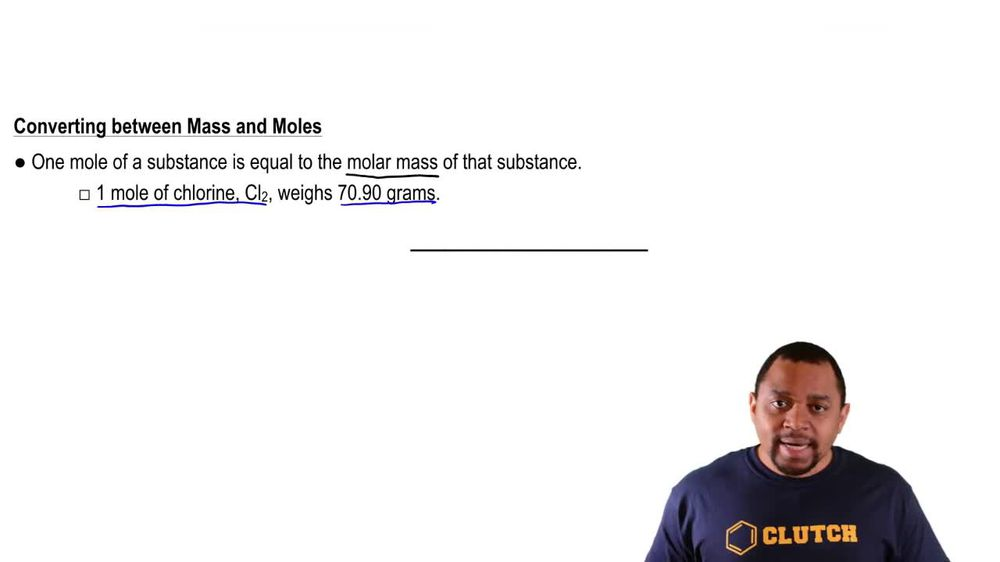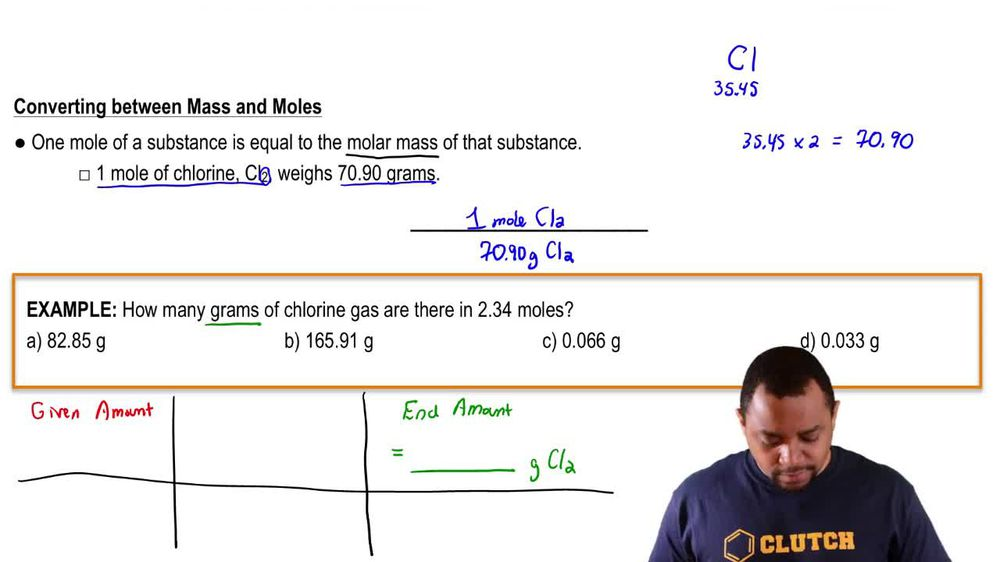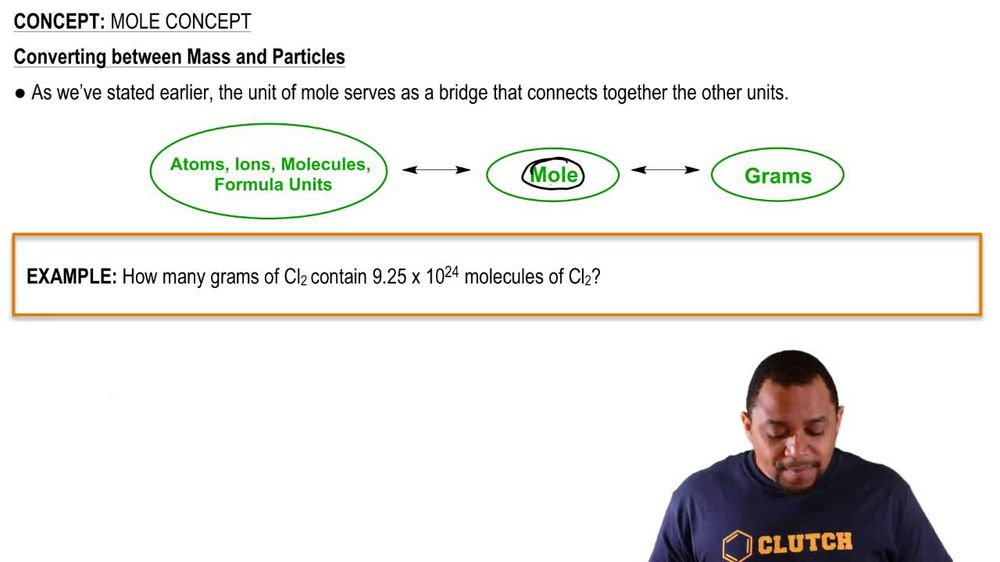Start typing, then use the up and down arrows to select an option from the list.
1. 2. Atoms & Elements2. Mole Concept# Mole Concept

by Jules Bruno
119 views
0
Now, in order to convert between moles and particles, we have to utilize what's called alpha god Rose number now, avocados Number says that one mole of a substance is equal to 6.22 times 10 to the particles. Now this value here of 6.22 times 10 to the 23. That is avocados number. And we're gonna say the term particles, it's just a general term that is used for ions, atoms, molecules or formula units. We talked about each of these four terms up above. If we want to just combine them all until one idea we can use the term particles. Now we're going to say here that one mole of a substance is equal to avocados number right. So that would mean that one mole off chlorine remember, chlorine is a dye atomic element in its natural form equals 6.22 times 10 to the 23 molecules of CL, too. Now we need to realize here that this could be a conversion factor because it's combining two different units together. So we can say here that one mole off the L two is 6.22 times 10 to the 23 molecules off cl, too. And remember, conversion factors weaken. Do the reciprocal where we flip it so we could say avocados. Number on top moles of CO two on the bottom, depending on if we want units to cancel out in a certain way. Now, before we move on to the next section, just let's investigate this conversion factor a little bit more. We have one mole of chlorine chlorine. Remember its natural form of cl two. Why are we using the term molecules, though? Remember the term molecules is used when we have ah, compound that has two or mawr non metals together. Chlorine is a non metal, and there are two of them, right, so that's why we're using the term molecules. That's why we're not using ions, atoms or formula units. It can't be an ion because it doesn't possess a positive or negative charge. It can't be an Adam. We'd only use the term Adam if it was just one chlorine by itself. We can't use the term formula unit because Formula Unit would mean that we need to have a metal connected to that chlorine. Because, remember, Formula Unit is when you have a metal and nonmetal together. So again, if we're trying to go between moles and particles, we have to utilize avocados number on. We're using the term molecules here because we have to non metals together.